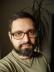## Perl Weekly Challenge 014: Van Eck and the US States

This week, there was more work to be done for some of us: in addition to the standard three challenges, there was one more from Neil Bowers. His suggestion for a challenge had been simplified, so he published the original specification.

## Van Eck’s Sequence

Let a0 = 0. Then, for n ≥ 0, if there exists an m < n such that am = an, take the largest such m and set an+1 = n − m; otherwise an+1 = 0.

I didn’t find a non-recursive formula for the sequence. I implemented a solution using an iterator: a subroutine that returns the next element of the sequence on each call.

``````#!/usr/bin/perl
use warnings;
use strict;

use Test::More;

my \$iterator = van_eck();

is_deeply([map \$iterator->(), 1 .. 19],
[0, 0, 1, 0, 2, 0, 2, 2, 1, 6, 0, 5, 0, 2, 6, 5, 4, 0, 5]);

done_testing();``````

Now, let’s try to rephrase the quoted definition of the sequence into Perl. It took me some time to do it right, although the idea is rather easy: For each number that appeared in the sequence, we need to remember the position of its last occurrence.

``````sub van_eck {
my %latest;
my (\$n, \$a_n) = (0, 0);
return sub {
my \$r = \$a_n;
my \$a_n_plus_1 = exists \$latest{\$a_n}
? \$n - \$latest{\$a_n}
: 0;
\$latest{\$a_n} = \$n++;
\$a_n = \$a_n_plus_1;
return \$r
}
}``````

It returns the 750_000th element of the sequence in one second on my machine.

## Traversing the US states

Using only the official postal (2-letter) abbreviations for the 50 U.S. states, write a script to find the longest English word you can spell.

Before I started coding, I decided the best way would be to read a dictionary word by word, checking for each one whether it could be generated from the postal codes. The dictionary is much longer than the list of the states, so we probably didn’t want to read it more than once.

As all the codes consist of 2 characters, we could skip all words of odd length. Each even length word would be split into character pairs, and if each pair is a valid postal code, we have a candidate.

``````#!/usr/bin/perl
use warnings;
use strict;
use feature qw{ say };

my %states;
@states{qw(AL AK AZ AR CA CO CT DE DC FL GA HI ID IL IN IA KS KY LA ME
MD MA MI MN MS MO MT NE NV NH NJ NM NY NC ND OH OK OR PA RI
SC SD TN TX UT VT VA WA WV WI WY)} = ();

my \$dictionary = '/usr/share/dict/british';
open my \$in, '<', \$dictionary or die \$!;
my @longest = ("");
while (my \$word = <\$in>) {
chomp \$word;
next if 1 & length \$word;

my \$uc_word = uc \$word;
my @pairs = \$uc_word =~ /\G(..)/g;

next if grep ! exists \$states{\$_}, @pairs;

next if length(\$word) < length \$longest;

if (length(\$word) == length \$longest) {
push @longest, \$word;
} else {
@longest = (\$word);
}
}
say for @longest;``````

On both `/usr/share/dict/{american|british}` provided by OpenSUSE Leap 42.3, the winners were calamondin and cascarilla (I hadn’t seen either of them before).

Neil Bowers posted Additional Perl Weekly Challenge 14 on Monday. To restrict the character combinations less, he proposed to use the initials instead of the postal codes, but only consider sequences of states that were adjacent.

At the beginning, the task didn’t specify whether a state could be visited more than once. This possibility was later prohibited, but I already had a solution ready, so I’ll show both of them.

To search for a path across the states, I used a recursive subroutine. It was implemented in a Prolog or Lisp way: the first parameter is the string we want to process, the second argument is the path travelled so far. In each step, we remove the first character from the first parameter and call the subroutine with the path extended by a state corresponding to it if possible. If the string is empty, we have found a path.

Some of the initials are of length 2, most of them are just single characters. That’s why there’s the loop over possible lengths. The first condition in the loop skips testing of the longer initials if only one character remains.

I also precomputed a hash mapping the initials to the states so I could find the path faster.

``````#!/usr/bin/perl
use warnings;
use strict;
use feature qw{ say };

use Cpanel::JSON::XS;

my \$json = <DATA>;

my \$data = Cpanel::JSON::XS->new->decode(\$json);
my %by_initials;
undef \$by_initials{\$data->{\$_}{initials}}{\$_} for keys %\$data;

my @longest = ([""]);
while (my \$word = <>) {
chomp \$word;
\$word = lc \$word;
if (my @path = path(\$word, [])) {
if (length \$word > length \$longest) {
@longest = ([\$word, \@path]);
} elsif (length \$word == length \$longest) {
push @longest, [\$word, \@path];
}
}
}

say "\$_->: ", map { map "[@\$_] ", @\$_ } @{ \$_-> } for @longest;

sub path {
my (\$rest, \$path) = @_;

return [\$path] if "" eq \$rest;

my @solutions;
for my \$length (1, 2) {
next if \$length > length \$rest;

my \$prefix = substr \$rest, 0, \$length;
next unless exists \$by_initials{\$prefix};

for my \$state (keys %{ \$by_initials{\$prefix} }) {
next if @\$path
&& ! grep \$state eq \$_,
push @solutions,
path(substr(\$rest, \$length), [ @\$path, \$state ]);

}
}
return @solutions
}
__DATA__

...``````

There were two winners (of length 8) on my machine: nonunion with a single possible path (NV OR NV UT NV ID OR NV) and matamata with 55 possible paths (go figure).

With the additional constraint that each state can be visited only once, we just need to modify the condition for `next` in the inner loop:

``````
next if @\$path
&& ((grep \$_ eq \$state, @\$path)
|| ! grep \$state eq \$_,I blog about Perl.